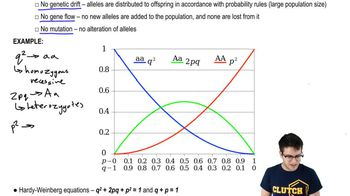Start typing, then use the up and down arrows to select an option from the list.
1:45 minutes
Problem 3
Textbook Question

# If the nucleotide variability of a locus equals 0%, what is the gene variability and number of alleles at that locus? a. gene variability=0%; number of alleles=0 b. gene variability=0%; number of alleles=1 c. gene variability=0%; number of alleles=2 d. gene variability>0%; number of alleles=2Verified Solution
This video solution was recommended by our tutors as helpful for the problem above.
157views